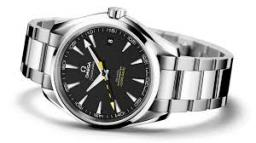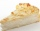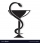# Minutes

Write as fraction in basic form which part of the week is 980 minutes.

Result

x = 7/72#### Solution:

$x=\frac{980}{7\cdot 24\cdot 60}=\frac{980}{10080}=\frac{7}{72}$We would be pleased if you find an error in the word problem, spelling mistakes, or inaccuracies and send it to us. Thank you!Tips to related online calculators
Need help calculate sum, simplify or multiply fractions? Try our fraction calculator.
Do you want to convert time units like minutes to seconds?

## Next similar math problems:Added together and write as decimal number: LXVII + MLXIV
• In fractionsAn ant climbs 2/5 of the pole on the first hour and climbs 1/4 of the pole on the next hour. What part of the pole does the ant climb in two hours?
• DoctorsIn the city operates 196 doctors. The city has 134456 citizens. How many citizens are per one doctor?
• Math classificationIn 3A class are 27 students. One-third got a B in math and the rest got A. How many students received a B in math?
• The resultHow many times I decrease the number 1632 to get the result 24?
• Fraction and a decimalWrite as a fraction and a decimal. One and two plus three and five hundredths
• Pizza 5You have 2/4 of a pizza and you want to share it equally between 2 people how much pizza does each person get?
• Mixed2improperWrite the mixed number as an improper fraction. 166 2/3
• Lengths of the poolMiguel swam 6 lengths of the pool. Mat swam 3 times as far as Miguel. Lionel swam 1/3 as far as Miguel. How many lengths did mat swim?
• CakesOn the bowl were a few cakes. Jane ate one-third of them, Dana ate a quarter of those cakes that remained. a) What part (of the original number of cakes) Dana ate? b) At least how many cakes could be (initially) on thebowl?
• Fractions 4How many 2/3s are in 6?
• ZdeněkZdeněk picked up 15 l of water from a 100-liter full-water barrel. Write a fraction of what part of Zdeněk's water he picked.
• Cake 71/3 of a cake shared with 4 people. What share of the whole cake has each people?
• A bakerA baker has 5 1/4 pies in her shop. She cut the pies in pieces that are each 1/8 of a whole pie. How many pieces of pie does she have?
• ChocolateChildren break chocolate first to third and then every part of another half. What kind got each child? Draw a picture. What part would have received if each piece have halved?
• IllnessPeter is ill and must take medication three times a day for one tablet. For how many days is a pack of 30 tablets enough for him, when on the third day he takes one tablet only in the morning and evening?
• Product of two fractionsProduct of two fractions is 9 3/5 . If one of the fraction is 9 3/7. Find the other fraction.